A ball is thrown upward with a certain speed. It passes through the same point at 3 second and 7 second from the start. The maximum height achieved by the ball is

1.  500 m

2.  250 m

3.  125 m

4.  450 m

Concept Questions :-

Uniformly accelerated motion
High Yielding Test Series + Question Bank - NEET 2020

Difficulty Level:

For the following acceleration versus time graph the corresponding velocity versus displacement graph is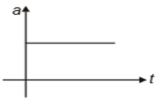1.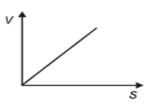2.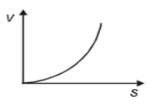3.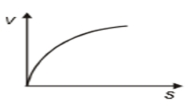4.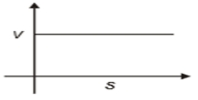Concept Questions :-

Uniformly accelerated motion
High Yielding Test Series + Question Bank - NEET 2020

Difficulty Level:

A particle is moving along the path y = ${\mathrm{x}}^{2}$ from x = 0 m to x = 2 m. Then the distance traveled by the particle is:

1.  4 m

2.

3.

4.

Concept Questions :-

Distance and displacement
High Yielding Test Series + Question Bank - NEET 2020

Difficulty Level:

Six particles situated at the corners of a regular hexagon of side 'a' move at constant speed v. Each particle maintains a direction towards the particle at the next. The time which the particles will take to meet each other is-

1.

2.

3.

4.

Concept Questions :-

Relative motion in 1-D
High Yielding Test Series + Question Bank - NEET 2020

Difficulty Level:

The displacement x of a particle moving in one dimension under the action of the constant force is related to time t by the equation , where x is in meters and t is in seconds. Find the displacement of the particle from t = 0 sec to t = 6 s.

1.  0

2.  12 m

3.  6 m

4.  18 m

Concept Questions :-

Non-uniform acceleration
High Yielding Test Series + Question Bank - NEET 2020

Difficulty Level:

The acceleration a (in ${\mathrm{ms}}^{-2}$) of a body, starting from rest varies with time t(in s) following the equation a = 3t+4. The velocity of the body at time t = 2s will be :

1. 10 ${\mathrm{ms}}^{-1}$

2. 18 ${\mathrm{ms}}^{-1}$

3. 14 ${\mathrm{ms}}^{-1}$

4. 26 ${\mathrm{ms}}^{-1}$

Concept Questions :-

Non-uniform acceleration
High Yielding Test Series + Question Bank - NEET 2020

Difficulty Level:

A stone falls freely from rest from a height h and it travels a distance $\frac{9h}{25}$ in the last second. The value of h is:

1. 145 m

2. 100 m

3. 122.5 m

4. 200 ms

Concept Questions :-

Uniformly accelerated motion
High Yielding Test Series + Question Bank - NEET 2020

Difficulty Level:

A point moves in a straight line under the retardation a${\mathrm{v}}^{2}$. If the initial velocity is u, the distance covered in 't' seconds is-

1.  $\mathrm{aut}$

2.

3.

4.

Concept Questions :-

Non-uniform acceleration
High Yielding Test Series + Question Bank - NEET 2020

Difficulty Level:

A particle is thrown upwards from ground. It experiences a constant resistance force which can produce retardation of 2 $m/{s}^{2}$. The ratio of time of ascent to the time of descent is:

(1) 1:1

(2) $\sqrt{\frac{2}{3}}$

(3) $\frac{2}{3}$

(4) $\sqrt{\frac{3}{2}}$

Concept Questions :-

Uniformly accelerated motion
High Yielding Test Series + Question Bank - NEET 2020

Difficulty Level:

Two men P & Q are standing at corners A & B of square ABCD of side 8 m. They start moving along the track with constant speed 2 m/s and 10 m/s respectively. The time when they will meet for the first time, is equal to: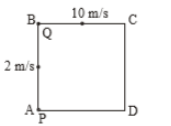(1) 2 sec

(2) 3 sec

(3) 1 sec

(4) 6 sec

Concept Questions :-

Relative motion in 1-D